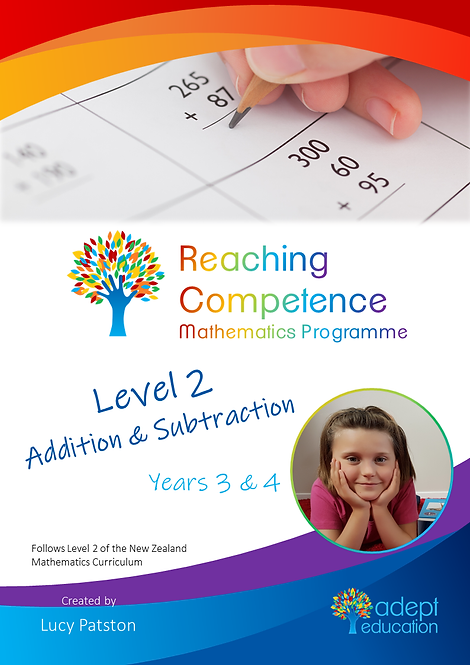`Level 2 Addition and Subtraction covers the addition and subtraction only learning intentions from the Linear Series for children aged about 7 to 9 years old (in Years 3 to 5). At this level, students are able to estimate reasonable answers to more difficult addition and subtraction equations, and they are becoming aware of the mental strategies needed to quickly compute equations with 2-digit numbers.  Get this 120-page activity booklet in PDF format, emailed immediately upon payment.`

# Level 2 Addition and Subtraction e-Copy

\$7.95Price
• Level 2 Addition and Subtraction - taken from the Reaching Competence Mathematics Programme.

At this level, students are able to estimate reasonable answers to more difficult addition and subtraction equations, and they are becoming aware of the mental strategies needed to quickly compute equations with 2-digit numbers.

You will also get: